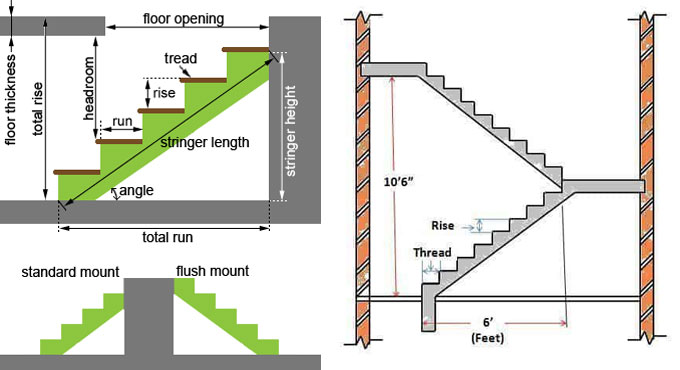# Calculating Staircase Slope and DimensionsStaircases are one of the most important parts of a building’s non-structural elements, since it is the primary way of providing access to the various floors of a building. As such, when constructing a staircase you will need to think about the structure in full 3D - the mere floor plan will not suffice. So, here is the way of calculating staircase slope and dimensions.

## Some Definitions of Stair

### Stair Run

The total horizontal distance traveled by a person while going through a staircase is called the stair run of that staircase. It is calculated from the face of the first riser to the last riser end.

### Stair Rise

The total vertical distance a staircase covers is called the stair rise of that staircase. It is measured between floor to floor, between the running surfaces. The stair rise of a staircase starts just before the first tread or step, and ends right after the ending stair tread at the top of the staircase.

The above two definitions play an important role in our discussion today about calculating staircase slope and dimensions, since these values depend directly on the stair run and stair rise. Figuring out the slope and dimensions of a staircase can be quite complicated, so we will take help of an example for better understanding, and learn on the job - so to speak.

### The Problem

Let us say, we already have the structural details of the two floors between which we will need to build the staircase. In general, architectural drawings will provide the FFL or Floor Finish Level, and the SSL or Structural Slab Level details already, in the elevation drawings.

Let us say, in our present example, the two levels are given as:

1. Landing level, at +1900 mm
2. Upper floor level, at +4000 mm

We will need to connect those two levels using a staircase. Follow the method described below carefully to figure out the slope and dimensions of the staircase and it will help you for the rest of your professional life.

## The Solution

### A. Calculating the Flight

First, let us find out the height of the staircase, that is, the stair rise.

We have two levels at +1900 mm and +4000 mm. Therefore, the stair rise should be:

Stair rise = difference between two levels
= 4000 -1900
= 2100 mm

Therefore, the staircase will be 2100 mm high. Or, the stair rise of the staircase is 2100 mm.

### B. Calculating Number of Steps

Here, we will figure out the number of steps in the staircase, or how many treads will be in this staircase. For that, we will need the height of each step. Generally, a safe height for each step of a staircase is taken at 150 mm; this is universally accepted as a comfortable and safe height for stair treads.

So we have,
Stair rise = 2100 mm
Step height = 150 mm
Therefore, no. of steps in the staircase = stair rise / step height
= 2100/150
= 14
So, we will need to provide 14 steps for the staircase in question.

### C. Calculating Tread Width

The next part is a bit tricky. The height of the space is divided by the number of steps that we have just obtained: 2100/14 = 150 mm height for each riser.

Now we need to estimate the width of the steps. For this, we will take the commonly assumed dimension of tread length, that is 300 mm. This is considered a safe tread length in most places.

So as per standard, we are providing 300 mm as tread length.

Number of Tread = Length of Stair/One number of Tread
= 3800/300
= 13

We know that: (T+2R=600)
so, T + 2x150 = 600mm
Or, T = 600-300 = 300mm (width of tread)

### D. Calculating the Slope

Finding out the slope of the staircase depends directly on the stair rise and stair run dimensions, which are 2100 mm and 3800 mm respectively. In order to work out our stair angle we can use a bit of basic trigonometry.

We have,
Total rise =1940 mm
Total Run = 3800mm
Tan = opposite/adjacent
= 1940 / 3800
= 0.51

Now, the inverse Tangent of 0.51 = 27 degrees

Therefore, the angle or slope of staircase = 27 degrees

As per IS 456, the slope or pitch of a stair should be between 25 degrees to 40 degrees, which our staircase complies to perfectly.

Hope the article was useful for you. Please let us know your thoughts and suggestions using the comments form below - we will love to hear from you! Happy learning.